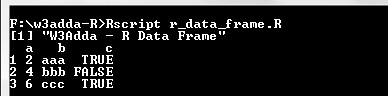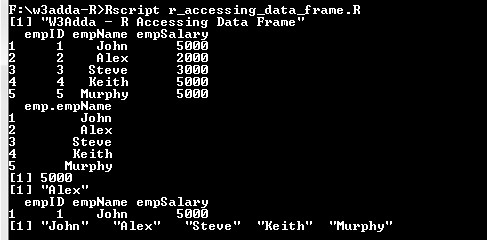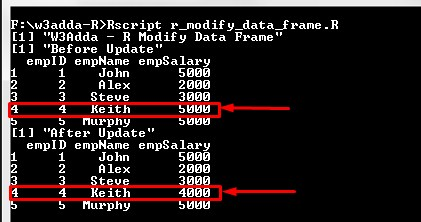# R Data Frames

In this tutorial you will learn about the R Data Frames and its application with practical example.

## R Data Frames

Data Frame is a data structure that contain list of vectors that is of equal length. Vectors in a data frame can be different data types i.e. numeric, character, factor, etc. It is a two dimensional data structure that us mainly used to store data tables.

Example:-

Here, df is a data frame containing three vectors a, b, c. When we run the above R script, we see the following output –

Output:-In Data Frame, each of the component form column and its contents form the rows.

## Creating a Data Frame

In R, a data frame is created using data.frame() function. The data.frame() function takes a vector input in order to create a factor.

Syntax:-

Above is the general syntax of data.frame() function, here

df:- It is collection of vector input that us being join as data frame, or it could be a matrix that can be converted to data frame.
stringsAsFactors:- It convert string to factor by default. To suppress this behavior, we can pass assign FALSE to it.

Let’s create a data frame using data.frame() function –

Example:-

Here, df is a data frame containing three vectors a, b, c. When we run the above R script, we see the following output –

Output:-## Accessing factor Elements

Elements in a data frame can be accessed same way as of list or matrix by passing row and column index value(s) in brackets [ ] separated with a comma you can access individual data frame element. However, individual column can be accessed using `[[` or `\$` operator.

Syntax:-

df:- Data frame name
row:- row index of the element, if not provided specified row elements will be fetched for all columns.
col:- column index of the element, if not provided specified column elements will be fetched for all rows.

Example:-

When we run the above R script, we see the following output –

Output:-## Modify Data frame Element

A data frame element can be modified by accessing element using above method and assigning it new value.

Example:-

Output:-## Data Frame rbind() and cbind() Function

The `rbind()` and `cbind()`function is used to add a row and column respectively to a data frame.

Example:-

Output:-## Delete Data frame Element

A data frame element can be deleted by simply accessing and assigning NULL value to it.

In this tutorial we have learn about the R Data Frames and its application with practical example. I hope you will like this tutorial.